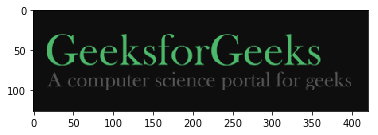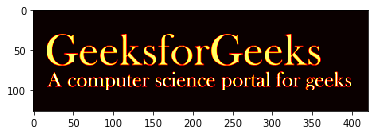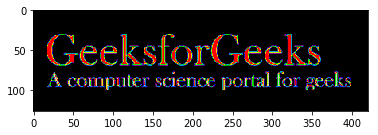Open in App
Not now

# Python | Visualizing image in different color spaces

• Difficulty Level : Easy
• Last Updated : 04 Jan, 2023

OpenCV (Open Source Computer Vision) is a computer vision library that contains various functions to perform operations on pictures or videos. It was originally developed by Intel but was later maintained by Willow Garage and is now maintained by Itseez. This library is cross-platform that is it is available on multiple programming languages such as Python, C++ etc.

Let’s discuss different ways to visualize images where we will represent images in different formats like grayscale, RGB scale, Hot_map, edge map, Spectral map, etc.

#### RGB Image :

RGB image is represented by linear combination of 3 different channels which are R(Red), G(Green) and B(Blue). Pixel intensities in this color space are represented by values ranging from 0 to 255 for single channel. Thus, number of possibilities for one color represented by a pixel is 16 million approximately [255 x 255 x 255 ].

 `# Python program to read image as RGB`` ` `# Importing cv2 and matplotlib module``import` `cv2``import` `matplotlib.pyplot as plt`` ` `# reads image as RGB``img ``=` `cv2.imread(``'g4g.png'``)`` ` `# shows the image``plt.imshow(img)`

Output :#### Gray Scale Image :

Grayscale image contains only single channel. Pixel intensities in this color space is represented by values ranging from 0 to 255. Thus, number of possibilities for one color represented by a pixel is 256.

 `# Python program to read image as GrayScale`` ` `# Importing cv2 module``import` `cv2`` ` `# Reads image as gray scale``img ``=` `cv2.imread(``'g4g.png'``, ``0``) `` ` `# We can alternatively convert``# image by using cv2color``img ``=` `cv2.cvtColor(img, cv2.COLOR_BGR2GRAY)`` ` `# Shows the image``cv2.imshow(``'image'``, img) `` ` `cv2.waitKey(``0``)         ``cv2.destroyAllWindows()`

Output :#### YCrCb Color Space :

Y represents Luminance or Luma component, Cb and Cr are Chroma components. Cb represents the blue-difference (difference of blue component and Luma Component). Cr represents the red-difference (difference of red component and Luma Component).

 `# Python program to read image``# as YCrCb color space`` ` `# Import cv2 module``import` `cv2`` ` `# Reads the image``img ``=` `cv2.imread(``'g4g.png'``)`` ` `# Convert to YCrCb color space``img ``=` `cv2.cvtColor(img, cv2.COLOR_BGR2YCrCb)`` ` `# Shows the image``cv2.imshow(``'image'``, img) `` ` `cv2.waitKey(``0``)         ``cv2.destroyAllWindows()`

Output :#### HSV color space :

H : Hue represents dominant wavelength.
S : Saturation represents shades of color.
V : Value represents Intensity.

 `# Python program to read image``# as HSV color space`` ` `# Importing cv2 module``import` `cv2`` ` `# Reads the image``img ``=` `cv2.imread(``'g4g.png'``)`` ` `# Converts to HSV color space``img ``=` `cv2.cvtColor(img, cv2.COLOR_BGR2HSV)`` ` `# Shows the image``cv2.imshow(``'image'``, img) `` ` `cv2.waitKey(``0``)         ``cv2.destroyAllWindows()`

Output :#### LAB color space :

L – Represents Lightness.
A – Color component ranging from Green to Magenta.
B – Color component ranging from Blue to Yellow.

 `# Python program to read image``# as LAB color space`` ` `# Importing cv2 module``import` `cv2`` ` `# Reads the image``img ``=` `cv2.imread(``'g4g.png'``)`` ` `# Converts to LAB color space``img ``=` `cv2.cvtColor(img, cv2.COLOR_BGR2LAB)`` ` `# Shows the image``cv2.imshow(``'image'``, img) `` ` `cv2.waitKey(``0``)         ``cv2.destroyAllWindows()`

Output :#### Edge map of image :

Edge map can be obtained by various filters like laplacian, sobel, etc. Here, we use Laplacian to generate edge map.

 `# Python program to read image``# as EdgeMap`` ` `# Importing cv2 module``import` `cv2`` ` `# Reads the image``img ``=` `cv2.imread(``'g4g.png'``)`` ` ` ` `laplacian ``=` `cv2.Laplacian(img, cv2.CV_64F)``cv2.imshow(``'EdgeMap'``, laplacian) `` ` `cv2.waitKey(``0``)         ``cv2.destroyAllWindows()`

Output :#### Heat map of image :

In Heat map representation, individual values contained in a matrix are represented as colors.

 `# Python program to visualize ``# Heat map of image`` ` `# Importing matplotlib and cv2``import` `matplotlib.pyplot as plt``import` `cv2`` ` `# reads the image``img ``=` `cv2.imread(``'g4g.png'``)`` ` `# plot heat map image``plt.imshow(img, cmap ``=``'hot'``) `

Output :#### Spectral Image map :

Spectral Image map obtains the spectrum for each pixel in the image of a scene.

 `# Python program to visualize ``# Spectral map of image`` ` `# Importing matplotlib and cv2``import` `matplotlib.pyplot as plt``import` `cv2`` ` `img ``=` `cv2.imread(``'g4g.png'``)``plt.imshow(img, cmap ``=``'nipy_spectral'``) `

Output :My Personal Notes arrow_drop_up